Email us to get an instant 20% discount on highly effective K-12 Math & English kwizNET Programs!

#### Online Quiz (WorksheetABCD)

Questions Per Quiz = 2 4 6 8 10

### MEAP Preparation - Grade 7 Mathematics1.58 Order of Operations: P E M D A S or B O D M A S

 When a numerical expression involves two or more operations, there is a specific order in which these operations must be performed. There are two ways of remembering the order in which mathematical operations have to be performed. Method 1: P E M D A S Letters in PEMDAS stand for: P - Parenthesis E - Exponents MD - Multiplication or Division from left to right AS - Addition or Subtraction from left to right The following phrase helps students remember the PEMDAS rule further: Please Excuse (My Dear) (Aunt Sally). Method 2: B O D M A S The letters in the word BODMAS stand for various operations. B - Stands for Bracket. O - Stands for "OF" or "Orders" (ie Powers and Square Roots, etc.) D - Stands for Division. M - Stands for Multiplication. A - Stands for Addition. S - Stands for Subtraction Example 1: 8 - 6 + 2 = 2 + 2 = 4 40 - 2(2+3) Correct : 40 - 2(5) = 40 - 10 = 30 Incorrect: 40 - 2(5) = 38(5) = 190 When there are two or more parenthesis, grouping symbols, you perform the inner most grouping symbol first. Example 2: 3 + 4 x 5 = 3 + 20 = 23 Note: 3 + 4 x 5 = 7 x 5 = 35 is wrong answer. Example 3: 2 + 2[ 1 + (5 - 1)2] =2 + 2[ 1 + (4)2] =2 + 2[ 1 + 8] =2 + 2[ 9] =2 + 18 =20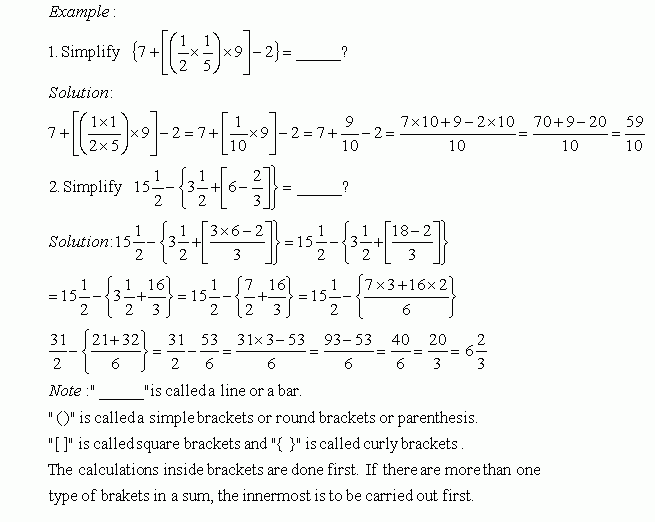Directions: Answer the following questions. Also write at least 5 examples of your own.
 Q 1: Solve the problem.222123 Q 2: Solve the problem.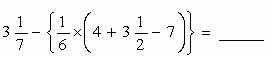4 6/602 5/803 5/84 Q 3: Solve the problem.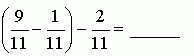7/116/116/22 Q 4: Solve the problem.2 1/31 2/32 2/3 Q 5: Solve the problem.131512 Q 6: Solve the problem.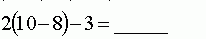102 Q 7: Solve the problem.11/912/910/9 Q 8: Solve the problem.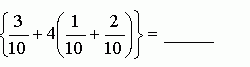2 1/41 2/31 1/2 Question 9: This question is available to subscribers only! Question 10: This question is available to subscribers only!